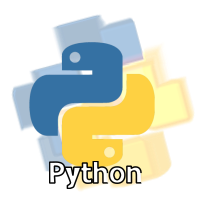# Y8

6e: Use simple arithmetic expressions in assignment statements to calculate values

Keywords:Test your self on these keywords and definitions using the games below or
KeywordDefinition
arithmetic expressionscombinations of variables, operators and numbers to calculate a value
arithmetic operatora mathematical symbol such as add (+), subtract (-), divide (/) or multiply (*)
assignment statementstoring a value into a variable
valuesdata such as a number
variablea named memory location which can store a value
Keyword games:
Resources: